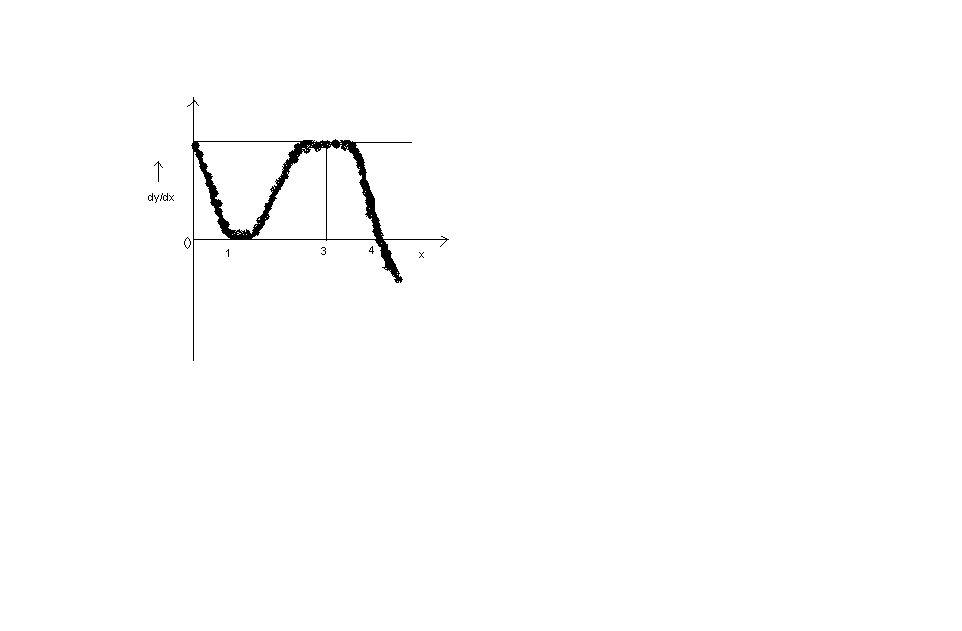# ParagraphThe diagram shows the graph of the derivative of a function y=f(x) for 0<=x<=5 with f(0)=0.

∫f(x)dx= [limits from 0 to 1]
a.-21/20 b.21/20
c.-1 d.none of these
Sir u said option b or d must be correct out of d two options......because f'(x) is increasing.....but answer is (a)..so how to do this????

•Anurag Ghosh ·

achcha sorry forgotto mention f'(0)=f'(3)=4.....

•f(0) is 0, f'(x)≥0 for all x between 0 to 1. So f(x) is always non-negative in that interval. Then how can the integral be negative?

•Anurag Ghosh ·

Hmmmm....dats wat i am not understanding the function is increasing one.....don't know where is d failasy??

•Anik Chatterjee ·

at x=1,it is an inflection point,because first derivative as well as second derivative are 0 at x=1,function must be increasing from x=0 to x=4(and having x=1 as an inflection point),then decreasing from x=4 to x=5 ...but i cant find out the integral..

•Anurag Ghosh ·

Dats wat is d problem wid everyone.....how to find the integral????....Manish sir could help us out...

•Anik Chatterjee ·

since there is a inflection point and hence assuming a cubic function,
let f(x)=ax3+bx2+cx..(there will be no constant term because f(0)=0)
now use f'(0)=f'(3)=4 & f'(4)=0 (use any three of them)...u will always get none of these as the answer(at least,i am getting so)..but,is (d) the correct option??

Anurag Ghosh ·

Anik how did u assume it to be a cubic equation???...And how is it related to point of inflexion????.

•Anik Chatterjee ·

i think i should have taken a fourth degree polynomial...since f'(x) has exactly 3 roots,f(x) must have atmost 4 roots...i thought that since x3 has a single inlection point...and since this eq. also has a single inflection point...this could be a cubic polynomial..

••